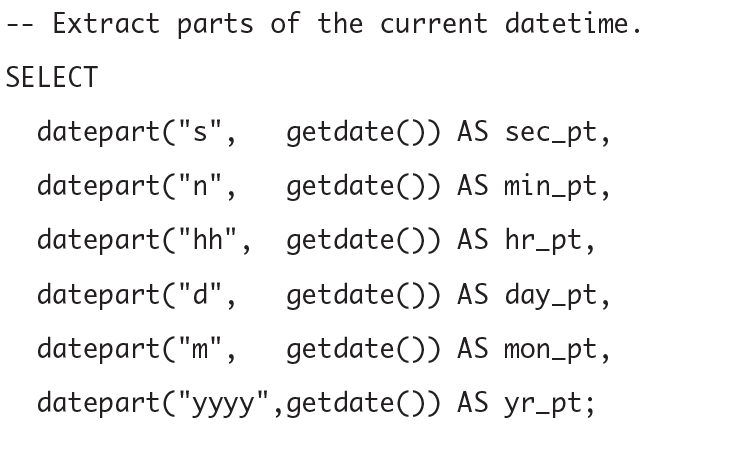SQL Functions   «Prev  Next»
 Lesson 15 The `DATEPART` function Objective Create a `SELECT` statement that will translate several date values by using date functions.

# SQL datepart() Function

Let us consider a table named Orders that has a column named OrderDate. Let's say you want to shift the OrderDate by 1 month. Here is how you would accomplish this using the DATEPART and DATEADD functions in SQL.
Firstly, the DATEPART function is used to get a part of a date such as year, month, day, hour, minute, etc. And, the DATEADD function is used to add an interval to a date and then return the date. The SQL statement you would use to achieve this is as follows:
```SELECT
OrderID,
OrderDate,
FROM
Orders;
```

In this statement:
1. DATEADD(month, 1, OrderDate) is the function that is adding 1 month to the OrderDate.
2. AS NewOrderDate is the alias you're giving to the new column that shows the translated date values.

In this query, you would replace 'Orders' and 'OrderDate' with the name of your table and column respectively.
This statement will return a result set with all the OrderID, the original OrderDate, and the OrderDate translated by one month. The translated date is returned in the NewOrderDate column.
Note that this is for SQL Server, the functions to add or subtract dates may vary slightly based on the specific SQL dialect you are using. In SQL dialects such as MySQL, functions like DATE_ADD or INTERVAL are used instead of DATEADD.
The `datepart() `function is easy to understand once you have worked with the other functions outlined in this module. `datepart()` will return the portion of the date you indicate. In other words, if you want to know what week of the year a given date represents, you can use `DATEPART` to determine that value.
The syntax for `datepart(n,m)` is:

```SELECT datepart(interval, date)
```

If you want to determine what week of the year 5/2/1998 falls in, you can do so with the following:
```SELECT datepart(week, '5/2/1998')
```

This will tell you that the date falls within the 18th week of the year. Of course, you can use the same interval indicators mentioned earlier, such as the day of the year, the month of the year, and so on.

The function datepart() extracts the specified part of a datetime.The function datepart() extracts the specified part of a datetime.

### Dates and Times

Support for date/time values is common in SQL products, although the details can vary from one product to another, largely because vendors implemented these data types before the SQL standard was developed. Various combinations of
1. dates,
2. times,
3. timestamps
4. , time intervals, and
5. date/time arithmetic
are generally supported. The SQL standard includes an elaborate specification for DATE, TIME, TIMESTAMP, and INTERVAL data types, including support for time zones and time precision (for example, tenths or hundredths of seconds).

## Date Function - Exercise

Complete the exercise to try out the date functions you have learned so far.
Date Function - Exercise